When I was in grad school, I wrote a paper on how to design Antenna Arrays using Window functions. I uploaded it to Academia.edu and forgot all about it. Once in a while I get an email from Academia.edu that someone searched for me. I got intrigued today and did a google search for “Antenna Arrays Windows” and was surprised to note that my paper is the second best match. Here is my shameless blog article to get that traffic to come to my site instead. If you prefer a PDF version of the paper, you can find it here.

####Abstract

The design of uniformly-spaced antenna arrays has a significant similarity to the design of FIR filters in signal processing and is illustrated first. This paper compares the effect of various windows on the shape of the radiation pattern of an antenna array. To illustrate the power of windows, we will design a sector pattern and a cosine pattern using various windows available namely Blackmann, Hanning, Hamming and the customizable Kaiser Window. Comparison is made on the radiation patterns produced whilst using each of the windows using the parameters of the generated pattern namely, beam width and side lobe level. To demonstrate the generality of the window design method, we see that there exists a window which gives an radiation pattern with all the side lobe levels having the same value which gives the same results as the Chebyshev Design Method for Uniformly Spaced arrays. Also, there is a window for Taylor Array Design Method.

###Introduction

The design of uniformly spaced arrays is extremely similar to the problem of FIR filter design. So, many methods that are used there can be used in antenna array design. One such method is the window based FIR filter design. We already know that the fourier series method used in antenna array design is also there in FIR filter design. We first explain the fourier series method briefly before going into the Window Method.

####Fourier Series Method Suppose it is desired to design a sector pattern of beamwidth 60

\begin{equation} \ f(\theta) = \left\{ \begin{array}{l l} 1 & \quad 0^\circ \leq \theta \leq 60 ^\circ \
0 & \quad elsewhere \end{array} \right.
\end{equation}

Design of array means finding the current excitations required on the array elements to give the required pattern. Since the currents and the radiation pattern are related through a fourier series, we can find the currents using that relation.

\begin{equation} SF_A(w) = \sum_{m=-N}^N i_m e^{j2\pi m \frac{d}{\lambda} w} \end{equation}

\begin{equation} SF_A(w) = \sum_{m=1}^N i_m e^{j\pi (2m-1) (\frac{d}{\lambda}) w} + \sum_{m=1}^N i_{-m} e^{j\pi (2m-1) (\frac{d}{\lambda}) w} \end{equation}

As we know, the Fourier Series expansion of a periodic signal $$x(t)$$ results in an infinite number of terms.

\begin{equation}c_n = \frac{1}{T_0} \int_{-T_0/2}^{T_0/2} x(t)e^{-jn2\pi f_0t} \,dt\end{equation}

\begin{equation}x(t) = \sum_{n=-\infty}^{\infty}c_ne^{jn2\pi f_0t}\end{equation}

But, we can have only finite number of elements in the array. Hence, we truncate. This could be viewed as applying a rectangular window to the actual fourier series expansion. For simplicity, we consider only the odd elements case. It could easily be extended to even elements case.

\begin{equation}SF_A(w) = \sum_{m=-\infty}^{\infty} i_m w_m e^{j2\pi m \frac{d}{\lambda}w}\end{equation}

where, $$w_m$$ is the window sequence which is defined as follows.

\begin{equation} w(m) = \left\{ \begin{array}{l l} 1 & \quad -N \leq m \leq N \
0 & \quad elsewhere \end{array} \right. \end{equation}

The effect of using the window is seen in the obtained approximate radiation pattern in the form of a main lobe width and side lobe levels. These are the signatures of the rectangular window function. Intuitively, the side lobes could be explained as due to the abrupt truncation of the fourier series coefficients.

The problem with using this window is that the ratio of the main lobe peak to the side lobe peak is always constant and doesn’t depend upon the number of elements we use. So, in an application where there is a need for a side lobe level which is significantly lesser than the main lobe peak, we cannot use this method to design the array.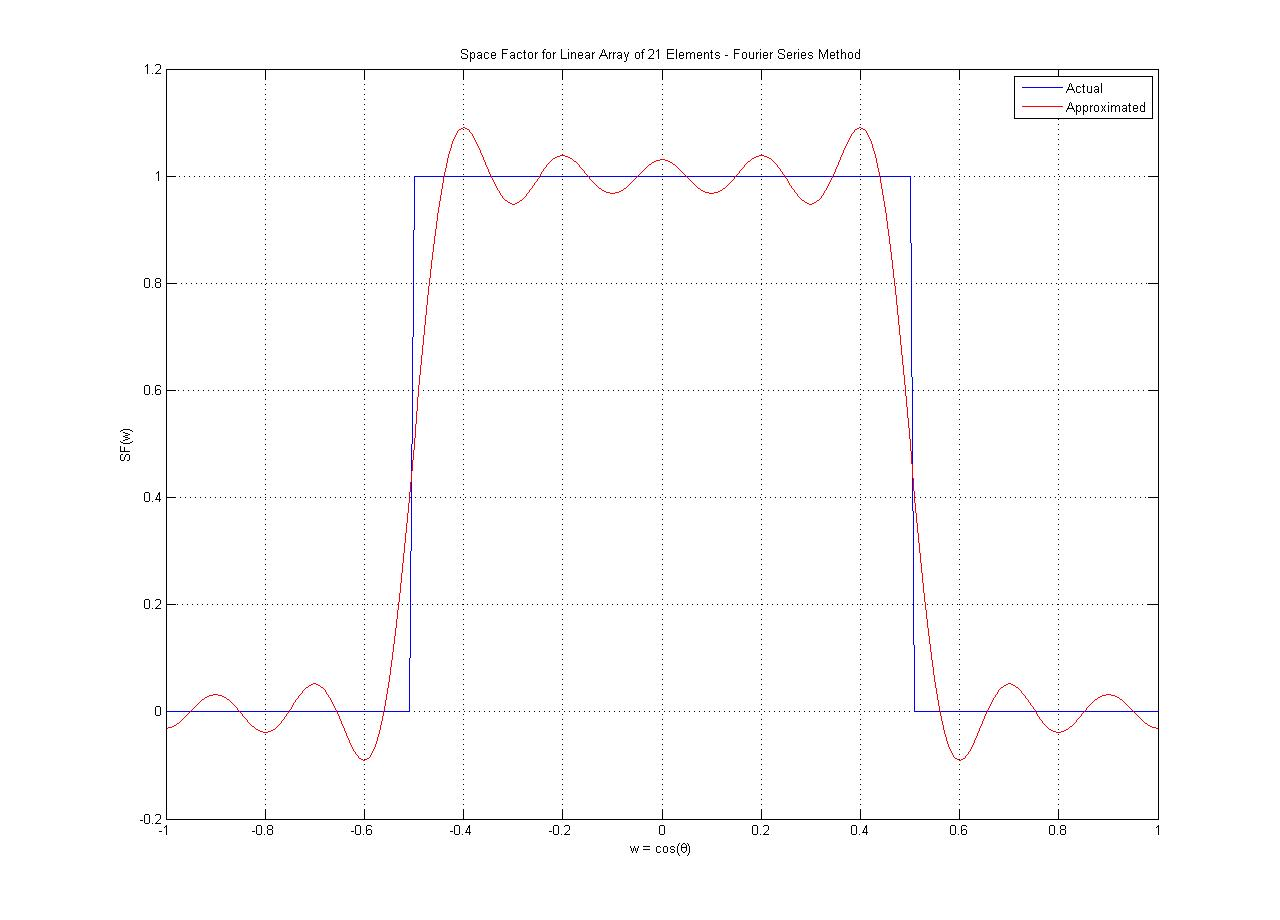#### Window based design

To reduce the side lobe levels in the generated pattern, we could smoothly taper the current distribution obtained from the Fourier Series Method using a properly chosen window. There are several windows available in the literature with each having some advantages over the other. Here, we will study the effect of using the following windows : Hann, Hamming, Blackman, Kaiser. These windows are defined by the following equations.

#####Hann \begin{equation} w(n) = \left\{ \begin{array}{l l} 0.5 - 0.5\cos(2\pi n/N), & \quad 0 \leq n \leq N \
0, & \quad otherwise \end{array} \right. \end{equation}

#####Hamming \begin{equation} w(n) = \left\{ \begin{array}{l l} 0.54 - 0.46\cos(2\pi n/N), & \quad 0 \leq n \leq N \
0, & \quad otherwise \end{array} \right. \end{equation}

#####Blackman \begin{equation} w(n) = \left\{ \begin{array}{l l} 0.42 - 0.5\cos(2\pi n/N) + 0.08\cos(4\pi n/N), & \quad 0 \leq n \leq N \
0, & \quad otherwise \end{array} \right. \end{equation}

Through a proper choice of the shape and the length of the window, we could control the resulting approximate radiation pattern. The windows defined in the above equations are fixed and offer very little in terms of control to the designer. This is where the Kaiser Window comes in. It gives one more parameter ($$\beta$$) to the designer which helps in controlling the shape of the window.

The compromise between the width of the main lobe and the area under the side lobe can be obtained by having a window function that is concentrated around 0 in frequency domain. This was considered in classic papers by Slepian et al. (1961). Kaiser(1966,1974) came up with a near-optimal window using modified first kind Bessel Function of the zeroth order. It is defined as follows

\begin{equation} w(n) = \left\{ \begin{array}{l l} \frac{I_0[\beta(1-[(n-\alpha)/\alpha]^2)^{1/2}]}{I_0(\beta)}, & \quad 0 \leq n \leq N \
0, & \quad otherwise \end{array} \right. \end{equation} where $$\alpha = N/2$$ and $$I_0(.)$$ is the Modified Bessel Function of zeroth order and first kind.

In figure 2, we have plotted the window function for various values of $$\beta$$ and a fixed value of M. In figure 3, we have plotted the fourier transform of the window functions. Finally, we have plotted the fourier transforms of windows with same $$\beta$$ parameter but different N parameter. For a more detailed reference on windows, refer to the paper by Harris.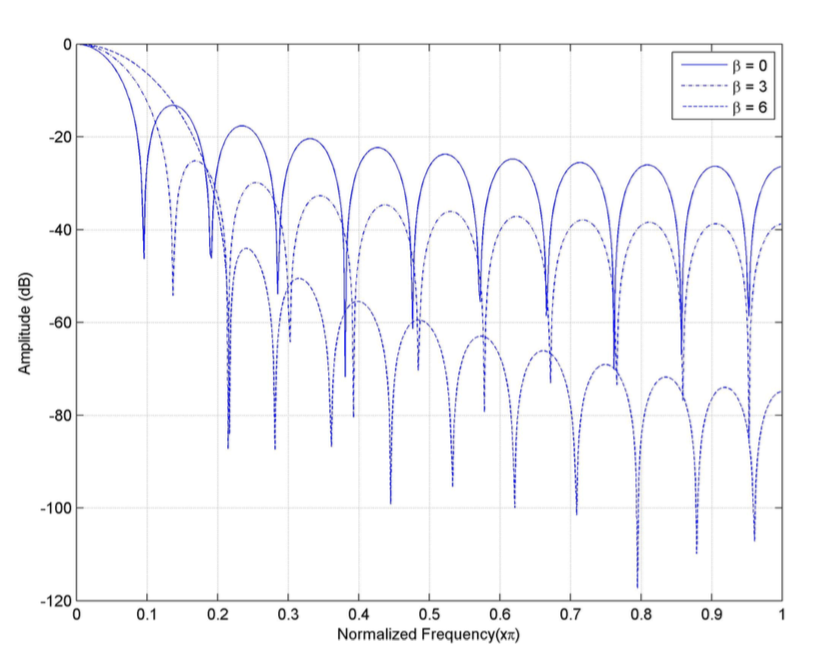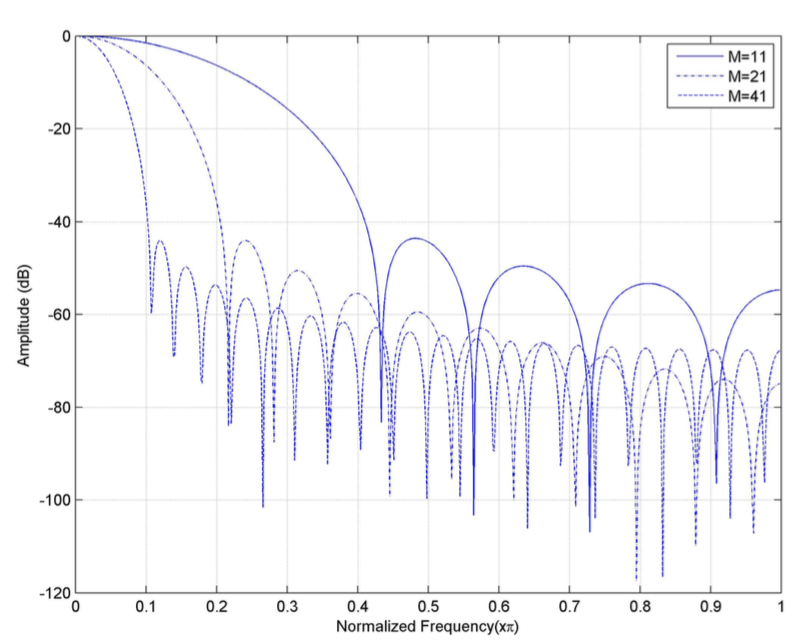Clearly, the parameter $$\beta$$ decides the side-Lobe Level. By keeping $$\beta$$ fixed and by increasing N, we could achieve a reduced main lobe width without any effect on the side lobe Level. Kaiser also came up with a pair of formulae that allows the designer to estimate the parameters M and $$\beta$$ for the required specification of peak approximate error. Peak Approximate Error as the name implies is the maximum error between the desired and the approximate response. Peak Approximate Error in a way specifies the maximum side lobe level $$A = -20log_{10}(\delta)$$. The empirical relation is as follows: \begin{equation} \beta = \left\{ \begin{array}{l l} 0.1102(A-8.7), & \quad A > 50, \
0.5842(A - 21)^{0.4} + 0.07886(A-21), & \quad 21 \leq A \leq 50, \
0.0, & \quad A < 21. \end{array} \right. \end{equation} Kaiser also determined that to achieve the required values of A and the $$\Delta \omega$$ (called the transition width), N must satisfy $$N = \frac{A-8}{2.285\Delta \omega}$$

Of particular importance are the special windows Chebychev and Taylor. The use of these windows result in a radiation pattern which are comparable to the results obtained using the Dolph’s Method  and Taylor’s Method . We say comparable since we don’t obtain the exact equiripple sidelobes since multiplying the Fourier Series Method current coefficients with the window is equivalent to convolving the Fourier transform of the window function with that of the approximate radiation pattern.

####Examples

First, we consider the example of a sector pattern as defined in equation (1) using various fixed windows. We have chosen an array having 21 elements to compare the performances of various windows. Clearly, we see a reduced side lobe level compared to the rectangular window output. But, the catch is we have an increased main lobe width. Performance of the sector pattern synthesis using window method is summarized in Table 1.

Window Beamwidth Sidelobe Level
Rectangular 68 -20.8
Hamming 85.6 -55.22
Hanning 81 -44.03
Blackman 116 -72.63

Table 1: Performance of various windows for Sector Pattern Synthesis

To see how the use of window affects a different pattern, we next consider the example of a cosine pattern  with main lobe maximum at 0◦ and a half-power beamwidth of 30◦. We see the performance while using various fixed windows is similar to that obtained for a Sector Pattern in terms of the Beam Width. But, the side lobe level performance is better for the Cosine Pattern synthesis. This can explained due to the smoothness of the pattern we started with in the first place. Performance of the cosine pattern synthesis using window Method is summarized in Table 2.

Window Beamwidth Sidelobe Level
Rectangular 68.1116 -39.2285
Hamming 85.6873 -63.4069
Hanning 81.0832 -61.1224
Blackman 116.4233 -94.7030

Table 2: Performance of various windows for Cosine Pattern Synthesis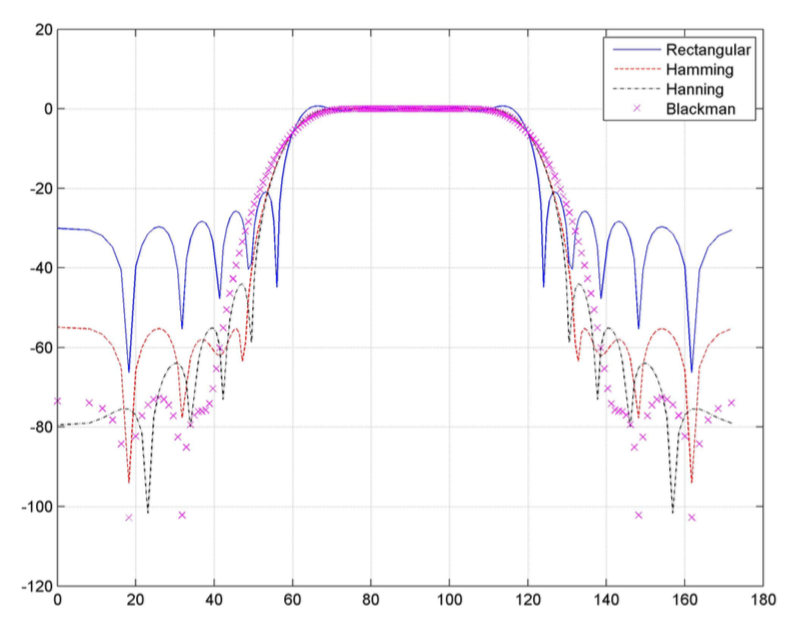####Kaiser window design of a sector pattern

Suppose it is desired to have a sector pattern with beam width of 60 degrees with a side lobe level of -26 dB and with a transition width of 0.2 (in normalized frequency). The design procedure is as follows :

1. Compute the required value of $$\beta$$ using equation (12) with $$A = -26 dB$$.
2. Compute the number of elements using equation (13) with $$\Delta \omega = 0.2$$.
3. Compute the currents using the Fourier series method.
4. Compute the window coefficients using the relation in (11).
5. Form the required currents by multiplying the currents obtained using the Fourier series method and the window function values.

For the given design specifications, we obtained a value of $$\beta = 1.5064$$ and M = 41. The approximate radiation pattern obtained while using these values are shown in figure 5. The Kaiser window design method provides a procedure to calculate the number of elements required in the array to achieve the specifications. To illustrate the improvement obtained by using Kaiser Window, we have plotted the radiation pattern of the array with size 41 while using a rectangular Window. Clearly, there is a 6 dB improvement.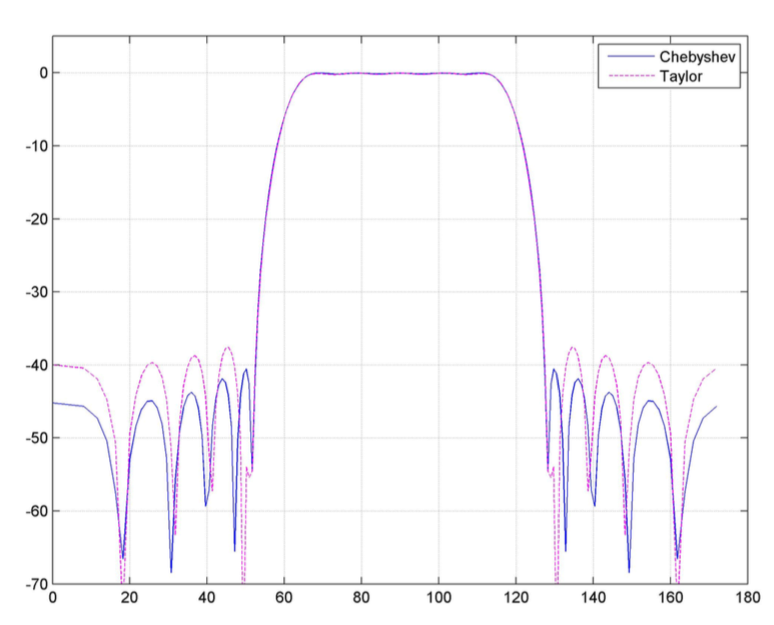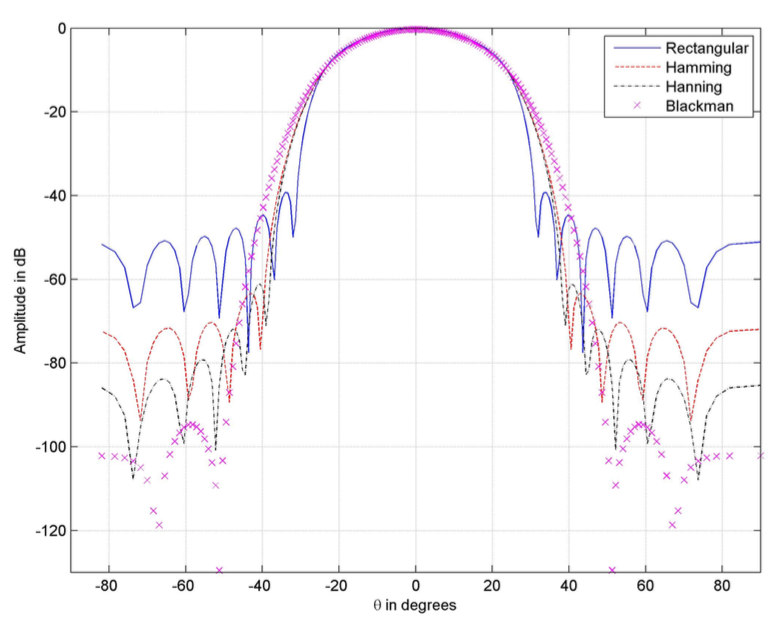####Conclusion

In essence, all the methods that are available for design of uniformly spaced array can be viewed as a special case of the window design method with a proper choice of window. We have demonstrated the generality by showing examples of Fourier Series Method and Chebyshev Method. Also, we have outlined a procedure for array design using Kaiser Window where we could specify the constraints in the required approximate pattern and could come up with a window function and the length of the array required to realize such a pattern.

####References

•  A. V. Oppenheim and R. W. Schafer, Digital Signal Processing. NJ: Prentice- Hall: Englewood Cliffs, 1975.
•  F. J. Harris, “On the use of windows for harmonic analysis with discrete fourier transform,” IEEE, vol. 66, 1978.
•  C.L.Dolph, “A current distribution for broadside arrays which optimizes the relationship between beamwith and sidelobe level,” IRE, vol. 34, pp. 335– 348, 1946.
•  T. T. Taylor, “Design of line-source antennas for narrow beamwidth and low sidelobes,” IRE Transactions Antennas Propagation, vol. AP-3, pp. 16–28, 1955.
•  D. H.Werner and A. J.Ferraro, “Cosine pattern synthesis for single and multiple main beam uniformly spaced linear arrays,” IEEE, vol. 37, no. 11, pp. 1480–1484, 1989.